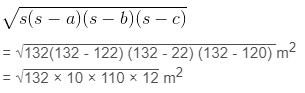Guru

# The triangular side walls of a flyover have been used for advertisements. The sides of the walls are 122 m, 22 m and 120 m (see Fig. 12.9). The advertisements yield an earning of ₹5000 per m2 per year. A company hired one of its walls for 3 months. How much rent did it pay? Q.2

• 1

I want to know the best answer of the question from Heron’s Formula chapter of class 9th ncert math. The question from exercise 12.1 of math. Give me the easy way for solving this question of  2 The triangular side walls of a flyover have been used for advertisements. The sides of the walls are 122 m, 22 m and 120 m (see Fig. 12.9). The advertisements yield an earning of ₹5000 per m2 per year. A company hired one of its walls for 3 months. How much rent did it pay?

Share

1. The sides of the triangle ABC are 122 m, 22 m and 120 m respectively.

Now, the perimeter will be (122+22+120) = 264 m

Also, the semi perimeter (s) = 264/2 = 132 m

Using Heron’s formula,

Area of the triangle ==1320 m2

We know that the rent of advertising per year = ₹ 5000 per m2

∴ The rent of one wall for 3 months = Rs. (1320×5000×3)/12 = Rs. 1650000

• 0